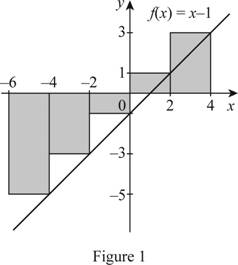# The Riemann sum for the function f ( x ) = x − 1 , − 6 ≤ x ≤ 4 with five subintervals and interpret the result using diagram.### Single Variable Calculus: Concepts...

4th Edition
James Stewart
Publisher: Cengage Learning
ISBN: 9781337687805### Single Variable Calculus: Concepts...

4th Edition
James Stewart
Publisher: Cengage Learning
ISBN: 9781337687805

#### Solutions

Chapter 5.2, Problem 1E
To determine

## To evaluate: The Riemann sum for the function f(x)=x−1,−6≤x≤4 with five subintervals and interpret the result using diagram.

Expert Solution

The Riemann sum for the function f(x)=x1,6x4 is -10.

The Riemann sum represents the net area of rectangles as shown in Figure 1.

### Explanation of Solution

Take the function as f(x)=x1,6x4.

The region lies between x=6 and x=4. So the limits are a=6 and b=4.

Consider the number of rectangles as n=5.

Find the width (Δx) using the relation:

Δx=ban (1)

Here, the upper limit is b, the lower limit is a, and the number of rectangles is n.

Substitute 4 for b, -6 for a and 5 for n in Equation (1).

Δx=4(6)5=105=2

Determine right end points using the relation:

xi=a+iΔx (2)

Calculate x1 using equation (2).

Substitute 1 for i and 2 for Δx in equation (2).

x1=6+(1×2)=4

Calculate x2 using equation (2).

Substitute 2 for i and 2 for Δx in Equation (2).

x2=6+(2×2)=2

Calculate x3 using equation (2).

Substitute 3 for i and 2 for Δx in Equation (2).

x3=6+(3×2)=0

Calculate x4 using equation (2).

Substitute 4 for i and 2 for Δx in Equation (2).

x4=6+(4×2)=2

Calculate x5 using equation (2)

Substitute 5 for i and 2 for Δx in Equation (2).

x5=6+(5×2)=4

Determine f(xi) using the equation:

f(xi)=xi1 (3)

Calculate f(x1) using equation (3).

Substitute 1 for i and -4 for x1 in the equation (3).

f(x1)=x11=41=5

Calculate f(x2) using equation (3).

Substitute 2 for i and -2 for x2 in the equation (3).

f(x2)=x21=21=3

Calculate f(x3) using equation (3).

Substitute 3 for i and 0 for x3 in the equation (3).

f(x3)=x31=01=1

Calculate f(x4) using equation (3).

Substitute 4 for i and 2 for x4 in the equation (3).

f(x4)=x41=21=1

Calculate f(x5) using equation (3).

Substitute 5 for i and 4 for x5 in the equation (3).

f(x5)=x51=41=3

The expression to find the Riemann sum of areas of n rectangles (Rn) is shown below:

Rn=i=1nf(xi)Δx=f(x1)Δx+f(x2)Δx+...+f(xn)Δx (4)

Here, the height of first rectangle is f(x1), the width is Δx,the height of second rectangle is f(x2), and the height of nth rectangle is f(xn).

Draw five rectangles using right endpoints as shown in Figure (1).Refer to Figure (1).

Take the height of first rectangle’s f(x1) value as -5, the height of second rectangle’s f(x2) value as -3, the height of third rectangle’s f(x3) value as -1, the height of fourth rectangle’s f(x4) value as 1, and the height of fifth rectangle’s f(x5) value as 3.

Substitute 5 for n, -5for f(x1), 2 for Δx, -3 for f(x2), -1 for f(x3), 1 for f(x4), and 3 for f(x5) in Equation (4).

R5=(5×2)+(3×2)+(1×2)+(1×2)+(3×2)=(10)+(6)+(2)+(2)+(6)=10

Therefore, the Reimann sum using right endpoints for n=5 is -10.

### Have a homework question?

Subscribe to bartleby learn! Ask subject matter experts 30 homework questions each month. Plus, you’ll have access to millions of step-by-step textbook answers!